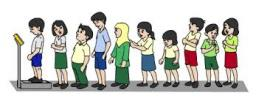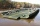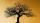# Boys and girls

There are 28 girls in the hall. 5/7 of all children are boys. How many children and how many boys are there?

Correct result:

c =  70
s =  98

#### Solution:

c+28 = s
5/7 s = c

c+28 = s
5/7•s = c

c-s = -28
7c-5s = 0

c = 70
s = 98

Our linear equations calculator calculates it.We would be pleased if you find an error in the word problem, spelling mistakes, or inaccuracies and send it to us. Thank you!Tips to related online calculators
Need help calculate sum, simplify or multiply fractions? Try our fraction calculator.
Do you have a linear equation or system of equations and looking for its solution? Or do you have quadratic equation?

## Next similar math problems:

• Simple equationSolve for x: 3(x + 2) = x - 18
• Unknown numberI think the number - its sixth is 3 smaller than its third.
• UN 1If we add to an unknown number his quarter, we get 210. Identify unknown number.
• One thirdIf 3/5 is 360, how much is 1/3?
• Unknown number 6Determine the unknown number, which is by 1.5 greater than its fourth.
• GivenGiven 2x =0.125 find the value of x
• New bridgeThanks to the new bridge, the road between A and B has been cut to one third and is now 10km long. How much did the road between A and B measure before?
• Equation with xSolve the following equation: 2x- (8x + 1) - (x + 2) / 5 = 9
• EqnSolve equation with fractions: 2x/3-50=40+x/4
• Eq-fracSolve the following equation with fractions: h + 1/3 =5/3
• FractionsThree-quarters of an unknown number are 4/5. What is 5/6 of this unknown number?
• Fraction + eqSolve following simple equation with fractions: -5/6(8+5b) = 75 + 5/3b
• FruitsAmy bought a basket of fruits 1/5 of them were apples,1/4 were oranges, and the rest were 33 bananas. How many fruits did she buy in all?
• PearsThere were pears in the basket, I took two-fifths of them, and left six in the basket. How many pears did I take?
• The bunsKate, Zofia and Peter Liked buns. Even today, their grandmother prepare their favorite meal. Katka eats 4 bunches, Žofia 3 and Petra eats 5 buns. Their grandmother said to them, "My inmate will you know how many buns I have been make today, if those you e
• Simple equation 324 = n • 27, solve for n
• Orchard3/5 of the trees in the orchard are apples, 1/3 are cherries and the remaining five trees are pear. How many trees are in the orchard?